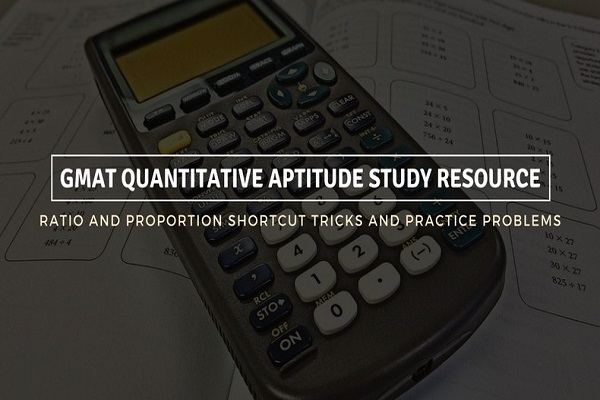# GMAT Quantitative Aptitude Study Resource: Ratio And Proportion Shortcut Tricks and Practice Problems

May 30, 2018Ratio and proportion have always played an important role in the GMAT quantitative aptitude section. These problems are usually scoring in nature and should be treated purely as word problems; nothing more, nothing less.

Here, in this post we have discussed this topic in depth for your reference.Ratio: A Brief Definition

The ratio of 2 quantities a and b in the same units, is represented by the fraction a/b. It can also be written as a : b.

In the ratio a : b, we call a as the 1st term (AKA antecedent) and b, the 2nd term (AKA consequent).

For e.g.

2:3=2/3

Here 2 is antecedent and 3 is consequent.

Point to remember: Division or multiplication of each term of a ratio by the same non-zero number doesn’t affect the ratio.

For example: 3:4 = 6:8 = 12:16

Proportion: A Brief Definition

Equality of 2 ratios is called proportion.

If a : b = c : d, we can write a : b :: c : d. Thus, we can say that a, b, c, and d are in proportion.

Here a and d are called extremes, and b and c are called the mean terms.

Product of means = Product of extremes.

Thus, a : b :: c : d

or, (b x c) = (a x d).

3. 4th, 3rd and Mean Proportion

i) 4th Proportion:

If a : b = c : d, then d is called the 4th proportion to a, b, c.

ii) 3rd Proportion:

a : b = c : d, then c is called the third proportion to a and b.

iii) Mean Proportion:

Mean proportion between a and b = √ab

Comparison of Ratios and Compounded Ratio

i) Comparison of Ratios:

When a:b > c:d, it means

ab>cd

ii) Compounded Ratio:

The compounded ratio of the ratios: (a : b), (c : d), (e : f) is (ace : bdf)

5. Important results of Ratio

i) Duplicate ratio of (a:b) = (a2:b2)

ii) Sub-duplicate ratio of (a : b) is = (√a:√b)

iii) Triplicate ratio of (a : b) = (a3:b3)

iv) Sub Triplicate ratio of (a : b) = (a1/3:b1/3)

v) If a/b = c/d,

then, a+b/a-b = c+d/c-d

6. Variation of Ratio

i) We can say that x is directly proportional to y, if x = ky for some constant k and then, we can write, x∝y

ii) We can say that x is inversely proportional to y, if xy = k for some constant k and then, we can write

x∝1/y

Shortcut Tricks

• Shortcut A: When A : B = m : n, and B : C = p : q. Then A : B : C = mp : pn :nq
• Shortcut B: When A : B = m : n, B : C = p : q, and C : D = r : s. Then A : B : C : D = mpr: npr : nqr : nqs

Practice ProblemsQuestion 1

In a box, there are a certain number of toy-blocks with alphabets P, Q, R and S written on them. The ratio of blocks P:Q:R:S is in the ratio 4:7:3:1. If the number of ‘P’ blocks is 50 more than the number of ‘R’ blocks, what is the number of ‘Q’ blocks?

Solution:

Let the number of blocks P, Q, R, S be 4x, 7x, 3x and 1x respectively

4x = 3x + 50

or, x = 50.

So the number of ‘Q’ blocks is 7*50 = 350.

Question 2

A certain recipe calls for 3 kilos of sugar for every 6 kilos of flour. If 60 kilos of this sweet has to be prepared, how much sugar is required?

Solution:

Let the quantity of sugar required be x.

3 kg of sugar added to 6 kg of flour adds up to a total of 9 kg of sweet.

3 kg of sugar is present in 9 kg of sweet. We’ll have to find out the quantity of sugar required for 60 kg of sweet. So the proportion should look something like this.

3/9 = x/60

or, x=20.

Therefore, 20 kg of sugar is required for 60 kg of sweet.

So that concludes the article for now. We will try to come up with more practice problems in the coming weeks but till then, goodbye!

**If you want take a quick peek at one of our previous articles on time and work, you can find it here.

***Looking for GMAT tutors in Dubai? This list can help.### MyPrivateTutor UAE

MyPrivateTutor is an online marketplace for all kinds of tutoring and learning services. We provide a platform to help learners find home tutors, online tutors, expert trainers,tutorial centers, training institutes and online tutoring schools. Learners can also find local classes, workshops, online courses and tutorials on a huge variety of topics. We operate in 12 countries worldwide and our community of learners, teachers and training businesses numbers over 200,000. We provide an opportunity for individual tutors and tutoring businesses to promote and grow their business.